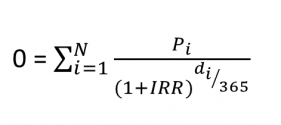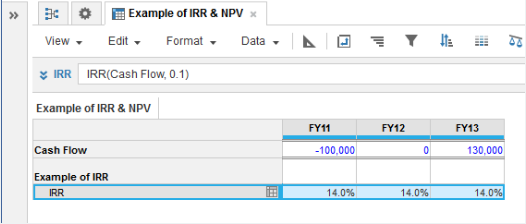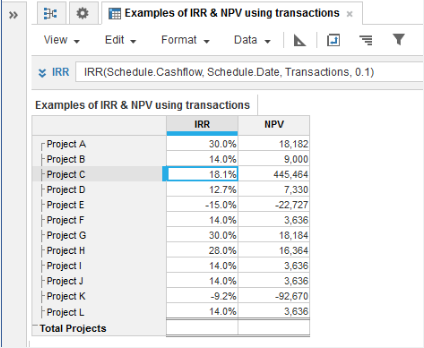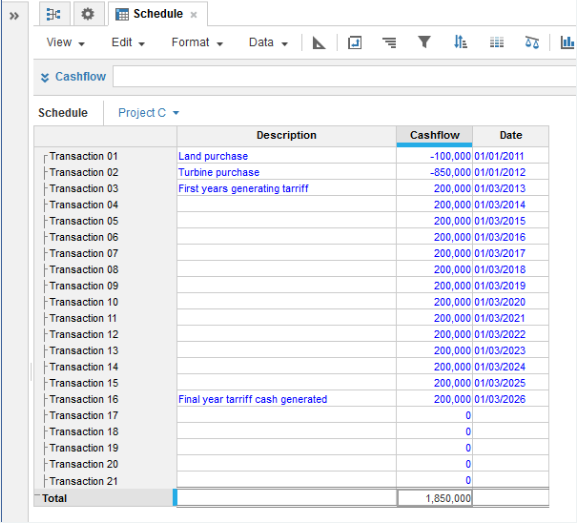1. Calculation functions
2. All Functions
3. Financial functions
4. IRRCalculates the internal rate of return of a series of future cash flows.

The second parameter is an optional guess at the IRR for use when multiple solutions exist.

To arrive at a solution there must be both negative and positive cash flows. Normally the initial investment is entered as a negative number for cash flow out and the returns entered as cash flow in. Since the formula for IRR is iterative, there may be multiple solutions. In that case a guess of the rate can be entered and the program will choose the solution closest to the guess.

For example

IRR(Cashflow,0.1)

sets an initial guess at 10% per period.

#### IRR using dates

Calculates the internal rate of return of a series of cash flows based on the transactions occurring on specified dates.

IRR(Cashflow, Dates, Transactions, Guess)

• Cashflow: Numeric cash values. Must have at least one negative and one positive value.
• Dates: The date of each transaction
• Transactions: The name of the list containing the schedule of transactions.
• Guess: A guess at the IRR. For example enter a guess as 0.1 for a 10% IRR. In the event of multiple solutions to the IRR formula, the program will choose the solution closest to the guess.

The IRR result does not require a time dimension.

IRR is the iterative solution to the equation below:• di is the number of days from the start of the first time period
• Pi is the payment in the ith period.
• N is the number of payments in and out over the entire timescale

## Syntax

IRR(c, [g])

where:

• c: Cash flow: line item
• g: Estimate of Rate: number (optional)

#### IRR using dates

IRR(c, d, t, [g])

where:

• c: Cash flow
• d: Dates
• t: Transaction List
• g: Estimate of Rate

## Format

Input Format Output Format

c: Number

d: Date

t: List item

g: Number

Number

## Arguments

The function uses the following arguments:

• c: Cash flow: Numeric line item, property, or expression
• d: Date: Hard-coded date, date variable, or line item
• t: Transaction List: List-formatted line item
• g: Estimate of Rate: Numeric line item, property, or expression (optional)

### Use IRR with the Users list

You can reference the Users list with the IRR function. However, you cannot reference specific users within the Users list as this is production data, which can change and make your formula invalid.

## Constraints

The function has the following constraints:

• Cash flow parameter must have at least one negative and one positive value.

## Example

IRR = IRR(Cash Flow, 0.1)Note that the IRR result does not require a timescale dimension: It is a single % result based on cash flows spanning the entire timescale.

### IRR Using Dates

In the example shown, a series of cash transactions and the dates are entered in the module named Schedule. This module has a list named Transactions as one of its dimensions. The IRR and NPV are calculated for each project.